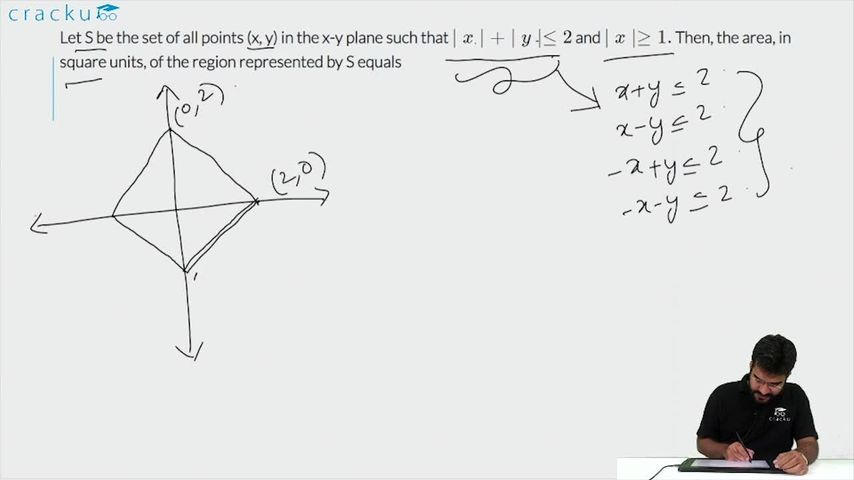Question 30

# Let S be the set of all points (x, y) in the x-y plane such that $$\mid x \mid + \mid y \mid \leq 2$$ and $$\mid x \mid \geq 1.$$ Then, the area, in square units, of the region represented by S equals

Solution

Sum of the area of region I and II is the required area.

Now, required area =  $$\ 4\times\frac{\ 1}{2}\times\ 1\times\ 1$$ = 2

### View Video Solution